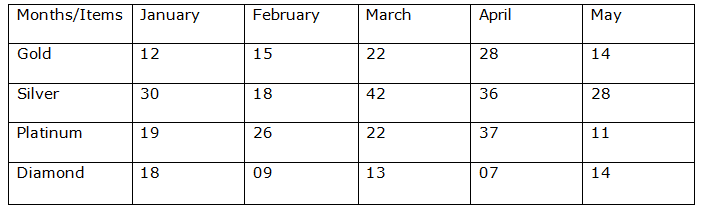# CWC/FCI Prelims 2019 – Quantitative Aptitude Questions (Day-35)

Dear Readers, Exam Race for the Year 2019 has already started, To enrich your preparation here we are providing new series of Practice Questions on Quantitative Aptitude – Section for CWC/FCI Exam. Aspirants, practice these questions on a regular basis to improve your score in aptitude section. Start your effective preparation from the right beginning to get success in upcoming CWC/FCI Exam.

[WpProQuiz 5842]

Directions (1 – 5): What value should come in place of question mark (?) in the following questions?

1) √1024 ÷ 42 × 7.5 + 63 + 279 =?

a) 510

b) 380

c) 260

d) 475

e) None of these

2) (4 × 2)4 ÷ (16 × 4)2 × (32 × 16)5 = 8?

a) 10

b) 6

c) 12

d) 15

e) None of these

3)  (5/3) of? – (2/3) of (12/17) of 3162 = 42 % of 850

a) 783

b) 857

c) 1107

d) 685

e) None of these

4) 25 % of x – 1664 ÷ 13 + 222 ÷ 6 = 67

a) 968

b) 745

c) 529

d) 632

e) None of these

5) √x × 13 – 26 % of 2650 – 12 × 28 = 15

a) 80

b) 3600

c) 60

d) 6400

e) None of these

Directions (6 – 10): Study the following information carefully and answer the given questions:

Following table shows the sales of different items in different months (in lakhs)6) The sales of gold in April and May is what percentage of the sales of diamond in March and April?

a) 265 %

b) 223 %

c) 210 %

d) 230 %

e) None of these

7) Difference between sales of gold and silver in all months together is approximately what percentage of difference between sales of silver and diamond in all months together?

a) 42 %

b) 32 %

c) 80 %

d) 70 %

e) 50 %

8) Find the average number of platinum sold in all the given months together (in lakhs)?

a) 16 lakhs

b) 26 lakhs

c) 18 lakhs

d) 23 lakhs

e) None of these

9) Find the ratio of total platinum sold in April and may to that of total silver sold in February and March?

a) 5:6

b) 4:5

c) 6:7

d) 5:3

e) None of these

10) The difference between average of diamond and silver sold in all months together is approximately what percentage more/less than the average of silver sold in all months together?

a) 60 % more

b) 60 % less

c) 40 % less

d) 40 % more

e) 25 % more

Direction (1-5) :

(32/16)*(15/2) + 216 + 279 =?

15 + 216 + 279 =?

? = 510

84 ÷ 642 × 5125 = 8x

84 ÷ 84 × 815 = 8x

84-4+15 = 8x

815 = 8x

x= 15

(5x/3) – (2/3) * (12/17) * 3162 = (42/100) * 850

(5x/3) – 1488 = 357

(5x/3)  = 1845

x = (1845*3)/5 = 1107

25 % of x – 1664 ÷ 13 + 222 ÷ 6 = 67

(25/100)*x – 128 + 37 = 67

(25/100)*x = 67 + 128 -37

(25/100)*x = 158

x = (158*100)/25

x = 632

√x × 13 – 26 % of 2650 – 12 × 28 = 15

√x × 13 – (26/100)*2650 – 336 = 15

√x × 13 – 689 – 336 = 15

√x × 13 = 15 +689 + 336

√x × 13 = 1040

√x = 1040/13 = 80

x = 802 = 6400

Direction (6-10) :

The sales of gold in April and May = 28 + 14 = 42 lakhs

The sales of diamond in March and April = 13 + 7 = 20 lakhs

Required % = (42/20) × 100 = 210 %

Difference between sales of gold and silver in all months together

= (12 + 15 + 22 + 28 + 14) – (30 + 18 + 42 + 36 + 28)

= 154 – 91 = 63 lakhs

Difference between sales of silver and diamond in all months together

= (30 + 18 + 42 + 36 + 28) – (18 + 9 + 13 + 7 + 14)

= 154 – 61 = 93 lakhs

Required % = (93 – 63)/93*100 = 32.25 % = 32 %

The average number of platinum sold in all the given months together

= > (19 + 26 + 22 + 37 + 11)/5

= > 115/5

= > 23 lakhs

Total platinum sold in April and may

= > 37 + 11 = 48 lakhs

Total silver sold in February and March

= > 18 + 42 = 60 lakhs

Required ratio = 48: 60 = 4: 5

The difference between average of diamond and silver sold in all months

= > (154 – 61)/5 = 93/5

Average of silver sold in all the months =154/5

Required % = (93/154) × 100 = 60.38% = 60 % less